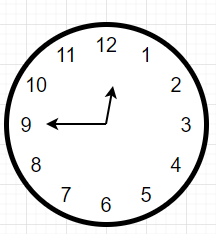# Program to find angle between hour and minute hands of a clock in C++?

Suppose we have two values hours and minutes. We have to find a smaller angle formed between the hour and the minute hand.

So, if the input is like hour = 12 minutes = 45, then the output will be 112.5To solve this, we will follow these steps:

• if h = 12, then set h := 0

• if m = 60, then set m := 0

• hAngle := 0.5 * (60h) + m

• mAngle := 6m

• ret := |hAngle - mAngle|

return minimum of ret and (360 – ret)Let us see the following implementation to get better understanding:

## Example

Live Demo

#include <bits/stdc++.h>
using namespace std;
class Solution {
public:
double solve(int h, int m) {
if(h == 12) h = 0;
if(m == 60) m = 0;
double hAngle = 0.5*((60 * h) + m);
double mAngle = 6 * m;
double ret = abs(hAngle - mAngle);
return min(360 - ret, ret);
}
};
main(){
Solution ob;
cout << (ob.solve(12, 45));
}

## Input

12, 45

## Output

112.5

Updated on: 10-Nov-2020

1K+ Views